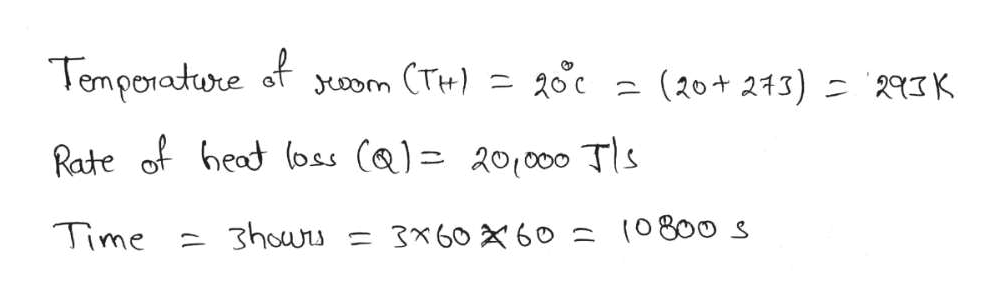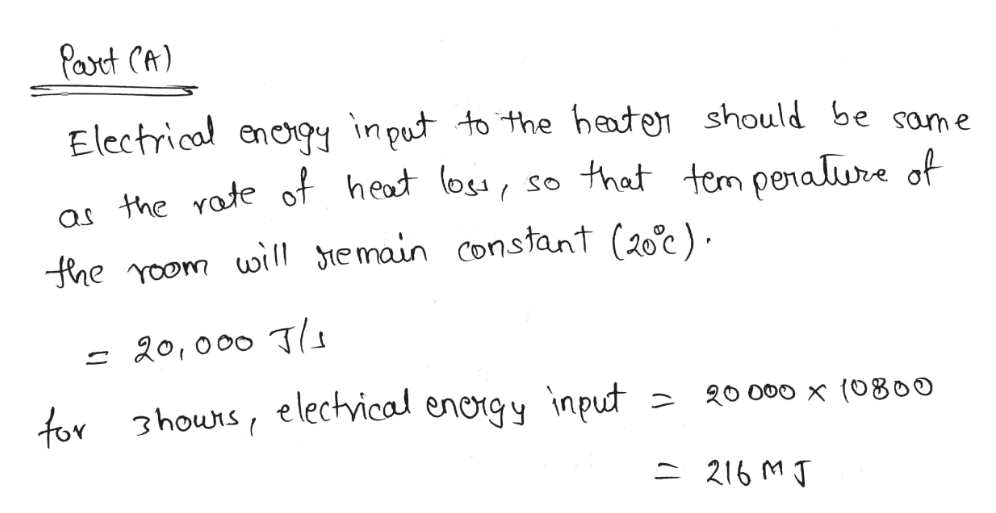# Consider three reservoirs that are TH = 473.2 K, TL = 293.2 K and TI = 277.2 K.  A Carnot heat engine can be reversed and used as either a heat pump or a refrigerator.  In the case of a heat pump the heat flow to the higher temperature reservoir is the desired output and it is used to heat a room or building.  In the case of the refrigerator the desired energy flow is the heat in from a region to be cooled.  For the heat pump or refrigerator, the work input is provided by electricity. Efficiency of the Carnot engine is 38%.For this information answer the following questions:A room is maintained at a constant temperature of 20 C and has a heat loss of 20,000 W.  Consider this room for a period of 3 hours.  The surroundings are at a temperature of TI.  In one option the room is heated using resistive heaters that are operated with electricity. a)Determine the electrical energy input to these heaters.b)Determine the efficiency of this heating system.c)Determine the entropy production for this this heating system.  In this case you should include the entropy production of the heat loss process to TI.d) The cost of electricity is \$0.237/kWh.  What is the cost to heat the room for 3 hours?

Question
24 views

Consider three reservoirs that are TH = 473.2 K, TL = 293.2 K and TI = 277.2 K.  A Carnot heat engine can be reversed and used as either a heat pump or a refrigerator.  In the case of a heat pump the heat flow to the higher temperature reservoir is the desired output and it is used to heat a room or building.  In the case of the refrigerator the desired energy flow is the heat in from a region to be cooled.  For the heat pump or refrigerator, the work input is provided by electricity. Efficiency of the Carnot engine is 38%.

For this information answer the following questions:

A room is maintained at a constant temperature of 20 C and has a heat loss of 20,000 W.  Consider this room for a period of 3 hours.  The surroundings are at a temperature of TI.  In one option the room is heated using resistive heaters that are operated with electricity.

a)Determine the electrical energy input to these heaters.

b)Determine the efficiency of this heating system.

c)Determine the entropy production for this this heating system.  In this case you should include the entropy production of the heat loss process to TI.

d) The cost of electricity is \$0.237/kWh.  What is the cost to heat the room for 3 hours?

check_circle

star
star
star
star
star
1 Rating
Step 1help_outlineImage TranscriptioncloseTenporatore oom (T 20c (20+ 213 23K Rate of heat loss (a) 201000 Tls Time 3houru 3x60 60 (0800 s fullscreen
Step 2help_outlineImage TranscriptionclosePart CA) Electrical encgy input to the haten should be as the Yote of heat (os, so that tem penaliure of ame fhe Yoom wil emain constant (20c) - 20,000 T 20 000 x (0800 for 3hours, elechvical enorgy input 216 M T fullscreen
Step 3

### Want to see the full answer?

See Solution

#### Want to see this answer and more?

Solutions are written by subject experts who are available 24/7. Questions are typically answered within 1 hour.*

See Solution
*Response times may vary by subject and question.
Tagged in

### Thermodynamics